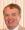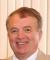# power model

## Polish translation: model funkcji potęgowej

GLOSSARY ENTRY (DERIVED FROM QUESTION BELOW)
 English term or phrase: power model Polish translation: model funkcji potęgowej Entered by:

 10:01 Nov 25, 2018
English to Polish translations [PRO]
Science - Mathematics & Statistics / statystyka kliniczna
 English term or phrase: power model PK variables over the dose ranges tested may be investigated using a power model.
 kgasPolandLocal time: 03:41
 model funkcji potęgowej Explanation:Propozycja. cccccc Funkcja potęgowa – funkcja postaci y = x^a, gdzie a jest daną liczbą rzeczywistą. https://pl.wikipedia.org/wiki/Funkcja_potęgowa cc Power functions Real functions of the form {\displaystyle f(x)=cx^{n}} {\displaystyle f(x)=cx^{n}} with {\displaystyle c\neq 0} c\neq 0 are sometimes called power functions.[citation needed] When {\displaystyle n} n is an integer and {\displaystyle n\geq 1} n\geq 1, two primary families exist: for {\displaystyle n} n even, and for {\displaystyle n} n odd. In general for {\displaystyle c>0} c>0, when {\displaystyle n} n is even {\displaystyle f(x)=cx^{n}} {\displaystyle f(x)=cx^{n}} will tend towards positive infinity with increasing {\displaystyle x} x, and also towards positive infinity with decreasing {\displaystyle x} x. All graphs from the family of even power functions have the general shape of {\displaystyle y=cx^{2}} {\displaystyle y=cx^{2}}, flattening more in the middle as {\displaystyle n} n increases. Functions with this kind of symmetry ( {\displaystyle f(-x)=f(x)} {\displaystyle f(-x)=f(x)}) are called even functions. When {\displaystyle n} n is odd, {\displaystyle f(x)} f(x)'s asymptotic behavior reverses from positive {\displaystyle x} x to negative {\displaystyle x} x. For {\displaystyle c>0} c>0, {\displaystyle f(x)=cx^{n}} {\displaystyle f(x)=cx^{n}} will also tend towards positive infinity with increasing {\displaystyle x} x, but towards negative infinity with decreasing {\displaystyle x} x. All graphs from the family of odd power functions have the general shape of {\displaystyle y=cx^{3}} {\displaystyle y=cx^{3}}, flattening more in the middle as {\displaystyle n} n increases and losing all flatness there in the straight line for {\displaystyle n=1} n=1. Functions with this kind of symmetry ( {\displaystyle f(-x)=-f(x)} {\displaystyle f(-x)=-f(x)}) are called odd functions. For {\displaystyle c<0} c < 0, the opposite asymptotic behavior is true in each case. https://upload.wikimedia.org/wikipedia/commons/thumb/2/2b/Po... https://en.wikipedia.org/wiki/Exponentiation#Power_functions
Selected response from:

Frank Szmulowicz, Ph. D.
United States
Local time: 21:41
 Dziękuję!4 KudoZ points were awarded for this answer

3model funkcji potęgowej
 Frank Szmulowicz, Ph. D.3 hrs   confidence:model funkcji potęgowej

Explanation:
Propozycja.

cccccc
Funkcja potęgowa – funkcja postaci y = x^a, gdzie a jest daną liczbą rzeczywistą.
https://pl.wikipedia.org/wiki/Funkcja_potęgowa

cc
Power functions
Real functions of the form {\displaystyle f(x)=cx^{n}} {\displaystyle f(x)=cx^{n}} with {\displaystyle c\neq 0} c\neq 0 are sometimes called power functions.[citation needed] When {\displaystyle n} n is an integer and {\displaystyle n\geq 1} n\geq 1, two primary families exist: for {\displaystyle n} n even, and for {\displaystyle n} n odd. In general for {\displaystyle c>0} c>0, when {\displaystyle n} n is even {\displaystyle f(x)=cx^{n}} {\displaystyle f(x)=cx^{n}} will tend towards positive infinity with increasing {\displaystyle x} x, and also towards positive infinity with decreasing {\displaystyle x} x. All graphs from the family of even power functions have the general shape of {\displaystyle y=cx^{2}} {\displaystyle y=cx^{2}}, flattening more in the middle as {\displaystyle n} n increases. Functions with this kind of symmetry ( {\displaystyle f(-x)=f(x)} {\displaystyle f(-x)=f(x)}) are called even functions.

When {\displaystyle n} n is odd, {\displaystyle f(x)} f(x)'s asymptotic behavior reverses from positive {\displaystyle x} x to negative {\displaystyle x} x. For {\displaystyle c>0} c>0, {\displaystyle f(x)=cx^{n}} {\displaystyle f(x)=cx^{n}} will also tend towards positive infinity with increasing {\displaystyle x} x, but towards negative infinity with decreasing {\displaystyle x} x. All graphs from the family of odd power functions have the general shape of {\displaystyle y=cx^{3}} {\displaystyle y=cx^{3}}, flattening more in the middle as {\displaystyle n} n increases and losing all flatness there in the straight line for {\displaystyle n=1} n=1. Functions with this kind of symmetry ( {\displaystyle f(-x)=-f(x)} {\displaystyle f(-x)=-f(x)}) are called odd functions.

For {\displaystyle c<0} c < 0, the opposite asymptotic behavior is true in each case.

https://en.wikipedia.org/wiki/Exponentiation#Power_functions

 Frank Szmulowicz, Ph. D.United StatesLocal time: 21:41Works in fieldNative speaker of:English,PolishPRO pts in category: 201Dziękuję!

Login or register (free and only takes a few minutes) to participate in this question.

You will also have access to many other tools and opportunities designed for those who have language-related jobs (or are passionate about them). Participation is free and the site has a strict confidentiality policy.

## KudoZ™ translation help

The KudoZ network provides a framework for translators and others to assist each other with translations or explanations of terms and short phrases.